#### You may also like### Hallway Borders

What are the possible dimensions of a rectangular hallway if the number of tiles around the perimeter is exactly half the total number of tiles?### Square Pegs

Which is a better fit, a square peg in a round hole or a round peg in a square hole?### Boxed In

A box has faces with areas 3, 12 and 25 square centimetres. What is the volume of the box?

# Giant Rubik's Cube

##### Age 11 to 14 ShortChallenge Level

Working out how many cubes are not visible
For the 3 by 3 by 3 Rubik's cube, the only cube that is not visible is the cube that is in the middle.

There will be a smaller cube inside the 12 by 12 by 12 Rubik's cube which is not visible. The side length of the smaller Rubik's cube will be 10 smaller cubes. This can be seen in the diagram below, where only one face of the 12 by 12 by 12 cube is shown.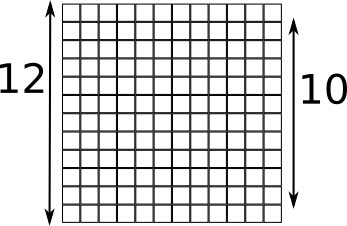This means that there will be a 10 by 10 by 10 cube that is not visible.

So the number of visible cubes must by 12$\times$12$\times$12$-$10$\times$10$\times$10 = 1728$-$1000 = 728.

Counting the cubes on each face
If we begin by counting the cubes visible on one of the faces, some of the cubes on the adjacent faces will also be counted, as shown.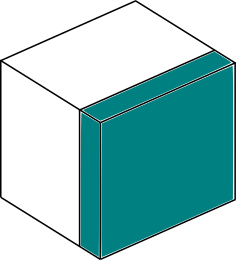12$\times$12 = 144 so 144 teal cubes have been counted. If we next count the face on top, coloured red in the diagram below, there are only 11$\times$12 = 132 more visible cubes, and some more of the cubes on adjacent faces will also be counted, as shown.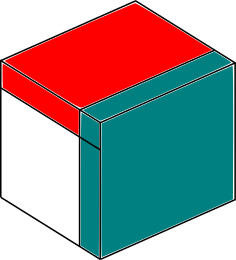The white face now only has 11$\times$11 = 121 new cubes to count, coloured yellow in the diagram below. In the diagram below, the cube has been rotated to show another uncounted face.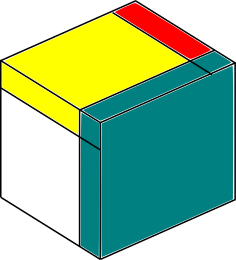This white face also has 11$\times$11 = 121 new cubes to count, coloured green in the diagram below. In the diagram below, the cube has been rotated to show another uncounted face. Notice that the red face is now at the bottom of the cube.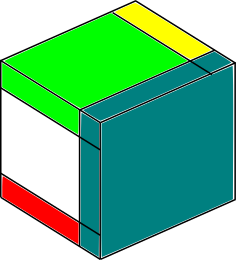This white face only has $10\times$11 = 110 new cubes to count, coloured purple in the diagram below.The diagram shows the remaining (back) face of the cube, with the red face still at the bottom.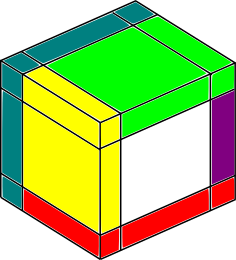There are 10$\times$10 = 100 cubes left to count on this face.

This gives a total of 144 + 132 + 121 + 121 + 110 + 100 = 728 visible cubes.
You can find more short problems, arranged by curriculum topic, in our short problems collection.